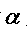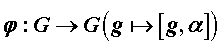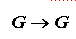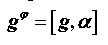# 有限生成剩余有限群的素数阶自同构Finitely Generated Residually Finite Groups with Automorphisms of Prime Order

• 全文下载: PDF(469KB)    PP.576-579   DOI: 10.12677/PM.2018.85076
• 下载量: 563  浏览量: 767   国家自然科学基金支持是有限生成剩余有限群G的素数p阶自同构，映射是满射，则G是幂零类至多为h(p)的幂零群，其中h(p)是与素数p有关的函数。特别地，如果是2阶自同构，那么G是交换群。

Letbe an automorphism of prime order p of a finitely generated residually finite group G. If the mapdefined byis surjective, then G is nilpotent of class at most h(p), where h(p) is a function depending only on p. In particular, ifis of order 2, then G is abelian.

1. 引言

Burnside  证明了：具有2阶无不动点自同构的有限群是奇阶交换群。这是有限群中的一个经典的结论。随后，Neumann  考虑了任意群的3阶无不动点自同构，得到了下面的结果。

2. 定理的证明及推论

$\underset{m}{\cap }{G}^{m}\le \underset{g}{\cap }{N}_{g}=1$

$\left[{g}_{1},{g}_{2},\cdots ,{g}_{h\left(p\right)+1}\right]\in {G}^{m}$

$\left[{g}_{1},{g}_{2},\cdots ,{g}_{h\left(p\right)+1}\right]\in \underset{m}{\cap }{G}^{m}=1$

$\left[{h}_{1},{h}_{2}\right]\in {N}_{g}$

$\left[{h}_{1},{h}_{2}\right]\in \underset{g}{\cap }{N}_{g}=1$

  Robinson, D.J.S. (1996) A Course in the Theory of Groups. 2nd Edition, Springer-Verlag, New York. https://doi.org/10.1007/978-1-4419-8594-1  Burnside, W. (1955) Theory of Groups of Finite Order. 2nd Edition, Dover Publications Inc., New York.  Neumann, B.H. (1956) Group with Automorphisms that Leave Only the Neutral Element Fixed. Archiv der Mathematik, 7, 1-5. https://doi.org/10.1007/BF01900516  徐涛, 刘合国. 有限秩的可解群的正则自同构[J]. 数学年刊, 2014, 35A(5): 543-550.  Xu, T. and Liu, H. (2016) Finitely Generated Torsion-Free Nilpotent Groups Admitting an Automorphism of Prime Order. Communications in Mathematical Sciences, 32, 167-172.  Thompson, J. (1959) Finite Groups with Fixed-Point-Free Automorphisms of Prime Order. Proceedings of the National Academy of Sciences of the United States of America, 45, 578-581. https://doi.org/10.1073/pnas.45.4.578  Higman, G. (1957) Groups and Rings Having Automor-phisms without Non-Trivial Fixed Elements. Journal of the London Mathematical Society, 64, 321-334. https://doi.org/10.1112/jlms/s1-32.3.321  Smelkin, A.L. (1968) Polycyclic Groups. Siberian Mathematical Journal, 9, 234-235. https://doi.org/10.1007/BF02196676  Hall, P. (1959) On the Finiteness of Certain Soluble Groups. Proceedings of the London Mathematical Society, 9, 595-622. https://doi.org/10.1112/plms/s3-9.4.595  Mal´cev, A.I. (1940) On the Faithful Representation of Infinite Groups by Matrices. Mat. sb., 8, 405-422.